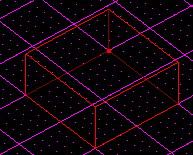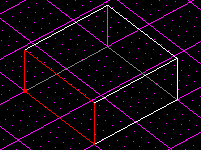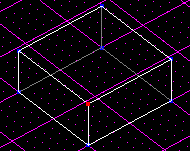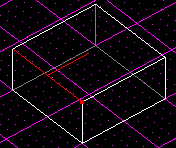# Terms

Author: 8t88

There are some essential terms you need to know while working with JK levels.

Sector - A sector is basically a room made up of vertices, edges, and surfaces.

Surface - A surface is one side of a sector, a flat shape (like a wall of a room), made up of vertices and edges.

Edge - An edge is where two surfaces meet and is represented by a line drawn between two vertices.

Vertex - A vertex is a single point, like a period on this page, that connects edges and makes angles possible.

Objects in red are defined below each image.SectorSurfaceVertexEdge